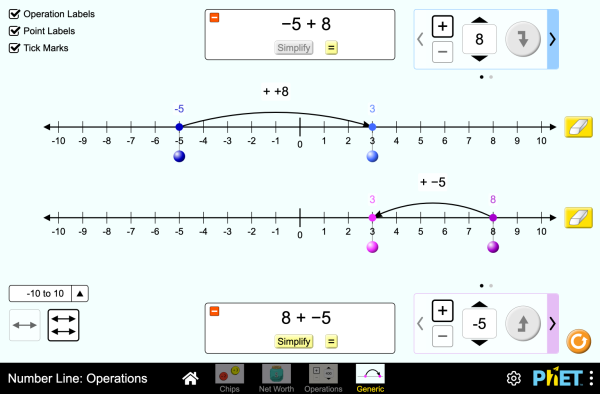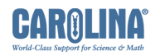# Number Line: Operations

Esta simulação não está traduzida neste idioma. Pode aceder à versão Inglesa mais abaixo.DescarregarIncorporar fechar Incorporar uma cópia em execução desta simulação Use este código HTML para incorporar uma cópia executável desta simulação. Pode alterar a altura e largura, da simulação que quer incorporar, modificando os atributos "largura" e "altura" no HTML. Incorpore uma imagem que lançará a simulação quando clicada
Clique para Iniciar
Use este código HTML para mostrar um panorama da simulação com as palavras "Clique para Iniciar". Operations Numero da Linha Integrais PhET é suportado pore educadores gostam de si.

### Tópicos

• Operations
• Numero da Linha
• Integrais

### Descrição

Explore operations with integers using an authentic context of net worth, then generalize with a number line. Experiment with adding and subtracting both positives and negatives, and make predictions about whether the total will be positive or negative.

### Amostra das Metas de Aprendizagem

• Represent addition and subtraction of integers on a horizontal number line
• Reason about addition and subtraction of integers in terms of number-locations
• Use logical necessity to reason that addition has the opposite effect as subtraction or that adding (or subtracting) a negative integer has the opposite effect as adding (or subtracting) a positive integer.
• Recognize and generate equivalence classes of integer sums and differences
• Show that a number and its additive inverse (opposite) have a sum of 0
• Using a net worth context, describe situations that have a positive sum or difference, negative sum or difference, and zero sum or difference
• View adding a negative as equivalent to subtracting a positive, and explain why this relationship makes sense in contexts such as net worth.
• View subtracting a negative as equivalent to adding a positive, and explain why this relationship makes sense in contexts such as net worth.
• Apply a number line model for addition and subtraction to new contexts.

#### Núcleo Comum - Matemática

7.NS.A.1
Apply and extend previous understandings of addition and subtraction to add and subtract rational numbers; represent addition and subtraction on a horizontal or vertical number line diagram.
7.NS.A.1a
Describe situations in which opposite quantities combine to make 0. For example, a hydrogen atom has 0 charge because its two constituents are oppositely charged.
7.NS.A.1b
Understand p + q as the number located a distance |q| from p, in the positive or negative direction depending on whether q is positive or negative. Show that a number and its opposite have a sum of 0 (are additive inverses). Interpret sums of rational numbers by describing real-world contexts.
7.NS.A.1c
Understand subtraction of rational numbers as adding the additive inverse, p - q = p + (-q). Show that the distance between two rational numbers on the number line is the absolute value of their difference, and apply this principle in real-world contexts.
7.NS.A.1d
Apply properties of operations as strategies to add and subtract rational numbers.
Versão 1.0.1

### Dicas para ProfessoresRevisão dos controlos das simulações, simplificação dos modelos, e instropeção ao pensamento do aluno ( PDF ).

Integer Addition and Subtraction Assessment Gillian Walker Secundário
Básico
Lab
TPC
Remote
Matemática
Basque Tudo EuskaraZenbaki-lerroa: eragiketak
Bosnian Tudo BosanskiBrojevna prava: Operacije
Chinese (Traditional) Tudo 中文 (台灣)Number Line: Operations_數線：數線的運算
Croatian Tudo hrvatskiBrojevna crta: Računske operacije
Danish Tudo DanskTallinje: Beregninger
Dutch Tudo NederlandsGetallijn: Bewerkingen
French Tudo françaisDroite numérique: opérations
German Tudo DeutschZahlenstrahl: Operationen
Greek Tudo ΕλληνικάΑριθμογραμμή: πράξεις
Hungarian Tudo magyarSzámegyenes - műveletek
Italian Tudo italianoRetta dei numeri: operazioni
Latvian Tudo LatviešuSkaitļu ass: Darbības
Maori Tudo MaoriRārangi Tau: Paheko
Polish Tudo polskiOś liczbowa: działania
Português (Brazil) Tudo português (Brasil)Reta Numérica: Operações
Serbian Tudo СрпскиБројевна права: Операције
Slovak Tudo SlovenčinaČíselná os: Operácie
Spanish Tudo españolRecta Numérica: OperacionesSpanish (Peru) Tudo español (Perú)Recta numérica: Operaciones
Turkish Tudo TürkçeSayı Doğrusu: İşlemler
Vietnamese Tudo Tiếng ViệtĐường thẳng số: Các phép toán

HTML5 sims can run on iPads and Chromebooks, as well as PC, Mac, and Linux systems on modern web browsers. If you are experiencing issues using an HTML5 sim on a supported platform, please disable any browser extensions.

iOS 12+ Safari

Android:
Not officially supported. If you are using the HTML5 sims on Android, we recommend using the latest version of Google Chrome.

Chromebook:
The HTML5 and Flash PhET sims are supported on all Chromebooks.
Chromebook compatible sims

Windows Systems: